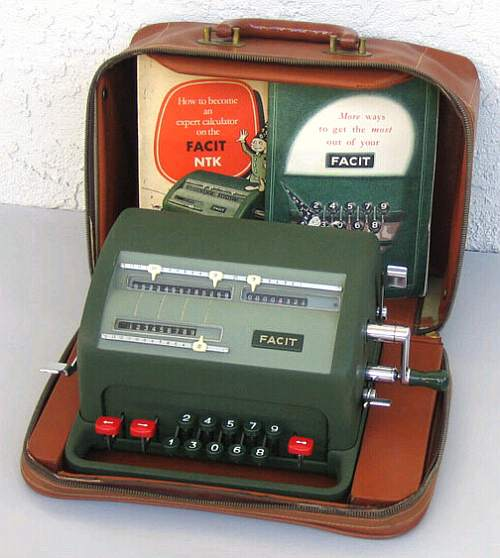previous <<==>> next

FACIT   NTK``` T E C H N I C A L D A T A of the Mechanical Calculator FACIT NTK *************************************************************************** with Booklets & Travel Case Designed by S. Bernadotte, Member of the Swedish Royal family Serial Number(s): 460769 (=Label outside) 347-126747 (=Engraving inside) Dimensions: (ca.) Width = 12 " / 30 cm Depth = 8 " / 20 cm Height = 6 " / 15 cm Weight: (ca.) 16 lbs / 7 kg Mechanics: 10-Keyboard (DALTON type) Serial-to-parallel conversion with Principle: Pin-Wheel / Sprossenrad Special: FACIT's Solution Functions: Add, Subtract, Multiply, Divide Registers: I Input = 9 Decimals II Counter = 8 Decimals III Arithmetic = 13 Decimals Manufacturer: FACIT Atvidaberg Sweden 1954 HOW TO REMOVE THE COVER: ************************ Remove the clearing levers (III = left) & (II = right) with a screw driver. Push CLIPS into the HOLES OF THESE (!) LEVERS ... and lift the cover simultaniously. H O W T O U S E the FACIT NTK ************************************* BASIC SETTINGS: =============== (A) Crank: ---------- For addition (+) the crank is turned clockwise; for subtraction (-) the crank is turned counter-clockwise. In the idle position the crank is DOWN & LOCKED. To make one or more turns with the crank, pull out the handle to unlock. When finished the turn(s), let the handle snap into lock again. The locked down position only makes other functions (ex. clearing) accessible. REMARK: Every started turn has to be finished completely! Accidentally started turns are correctible somehow. (B) Clearing Registers: ----------------------- Pulling down the LEFT lever will clear the arithmetic unit (III). Pulling down the RIGHT lever behind the crank will clear counter (II). Pulling up the RIGHT lever in front of the crank (with thumb on lever and index finger on crank axle) will clear and reset the input unit (I). REMARKS: It is intended to clear registers (I) & (II) with one grip. Prior to each task, reg.(I),(II)&(III) have to be cleared. (C) Shifting the Input Unit with the Entered Number: ---------------------------------------------------- Left of the number keys, the RED KEY-PAIR let the input unit STEP left [<] or right [>]. The actual position is marked with a red arrow-pair ref. to reg. (III) & (II). Right of the number keys, the RED [<-o] KEY let the input unit JUMP to the most left position - but the arrow-pair stops at pos. 8 of the counter - and the input will be filled up with zeros. (D) Counting Direction: ----------------------- After clearing the counter unit (II), it depends of the first crank turn (clockwise or counter-clockwise), that the counter will go either (+) or (-). In case of (+) the counter & arithmetic unit are working in the same; in case of (-) both are working in opposite direction. This is shown with a RED INDICATOR at the right of the counter unit. ADDITION & SUBTRACTION: ======================= Example: 123 + 45 - 6 = 162 ADD: Enter the first number (123) into input unit. Make a positive (clockwise) turn with the crank to transfer the number into arithmetic unit. The counting unit displays the figure 1. Clear the input unit. Enter the second number (45). Make a positive (clockwise) turn with the crank to add the number. The arithmetic unit displays the intermediate sum (168) and the counting unit displays the figure 2. SUBTRACT: Clear the input unit. Enter the third number (6). Make a negative (counter-clockwise) turn with the crank. The arithmetic unit displays the result (162) and the counting unit is decreased by 1. REMARK: NEGATIVE RESULTS are displayed in the arithmetic unit as the COMPLEMENT of the next higher 10, 100, 1000, ... Example: -12 = 99...9988 MULTIPLICATION: =============== Example: 123 x 45 = 5535 Enter the multiplicand (123) into input unit. Make positive (clockwise) turns with the crank, until the second figure of the multiplicator (5) will appear in the 1st position of the counter unit. Move the input unit with the STEP [<] key to position 2. Repeat making positive turns with the crank, until the first figure of the multiplicator (4) appears in the 2nd position of the counter unit. The multiplication is done: The multi- plicand (123) stays in the input unit, the multiplicator (45) is in the counter and the result (5535) is in the arithmetic unit. DIVISION: ========= Example: 22 : 7 = 3.1428571 Remainder 3 Division requires 3 steps: (A) To Set the Dividend into Arithmetic Unit: ----------------------------------------- Enter the dividend (22) into input unit. Press the JUMP [<-o] key. Make a positive (clockwise) turn with the crank to transfer the input into arithmetic unit. (B) To Set the Divisor into Input Unit: ----------------------------------- Clear input and counter unit with one grip. Enter the divisor (07) into input unit. Press the JUMP [<-o] key. (C) To Divide: ---------- Make negative (counter-clockwise) turns with the crank (now the red indicator is on) until the arithmetic unit shows an "underflow". Make one positive (clockwise) turn with the crank. Move the input unit with the STEP [>] key one position to the right. Make negative turns again ... and repeat this procedure until the required number of decimals are calculated... The result (3.1428571) is in the counter unit, and the remainder (3) is in the arithmetic unit. The divisor (7) stays in the input unit, therefore an additional decimal can be estimated... ( 4, cause 4 x 7 = 28). REMARKS: ======== This calculator cames in a travel case with two booklets: A manual (28 p.) "How to become an expert calculator on the FACIT NTK" and with exercises "More ways to get the most out of your FACIT" (52 pages with covers). impressum: *************************************************************************** © C.HAMANN http://public.beuth-hochschule.de/~hamann 08/24/07 ```